# Electronics and Communication Engineering - Exam Questions Papers

36.
In analog signal given below is sampled at such a rate that the samples of signal can be given to a collection of buffers having capacity of either 24 samples/sec. or 25 samples/sec. The sampling rate is minimum such that it fully utilizes the collection of both types of buffers m(t) = 2 sin 480pt + 3 sin 720 pt
The folding (maximum) frequency is
600 Hz
300 Hz
1200 Hz
2400 Hz
Explanation: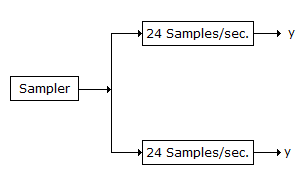LCM of 24 and 25 is 600 which will give maximum utilization of the buffers

Sampling frequency is 600

Folding frequency = 600/2 = 300.

37.
The system with given T.F.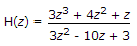to be stable will be
causal
anticausal
both sided
non causal
Explanation: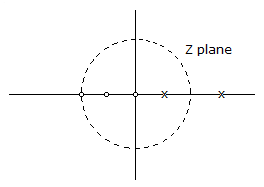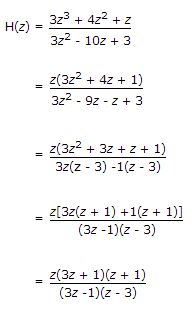Pole zero plot diagram is ROC should contain unit circle. Hence system is noncausal.

38.
For a Gaussian process, auto-correlation also implies
statistical dependence
statistical independence
ergodic process
none of the above
Explanation:

Statistical independence:

Suppose that Aj and Bk are the possible outcomes of two successive experiments or the joint outcome of a single experiment.

And suppose that it turns out that the probability of the occurrence of outcome Bk simply does not depend at all on which outcome Aj accompanies it.

Then we say that the outcomes Aj and Bk are independent.

Auto correlation also implies comparison of two signals of same source.

Hence for random Gaussian process. Auto correlation also implies statistical independence.

39.
The eigen values of a skew symmetric matrix are
always zero
always pure imaginary
either zero or pure imaginary
always real
Explanation:

For a real skew symmetric matrix the non-zero eigen values are all pure imaginary and thus occurs in complex conjugate pair.

40.
An LTI system having transfer function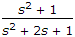and input x(t) = sin (t + 1) is in steady state. The output is sampled at a rate ωs rad/s to obtain the final output {y(k)}. Which of the following is true?
y(.) is zero for all sampling frequencies ωs
y(.) is nonzero for all sampling frequencies ωs
y(.) is nonzero for ωs > 2, but zero for ωs > 2
y(.) is zero for ωs > 2 but nonzero for ωs > 2
ω = 1,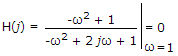Thus output is zero for all sampling frequencies.